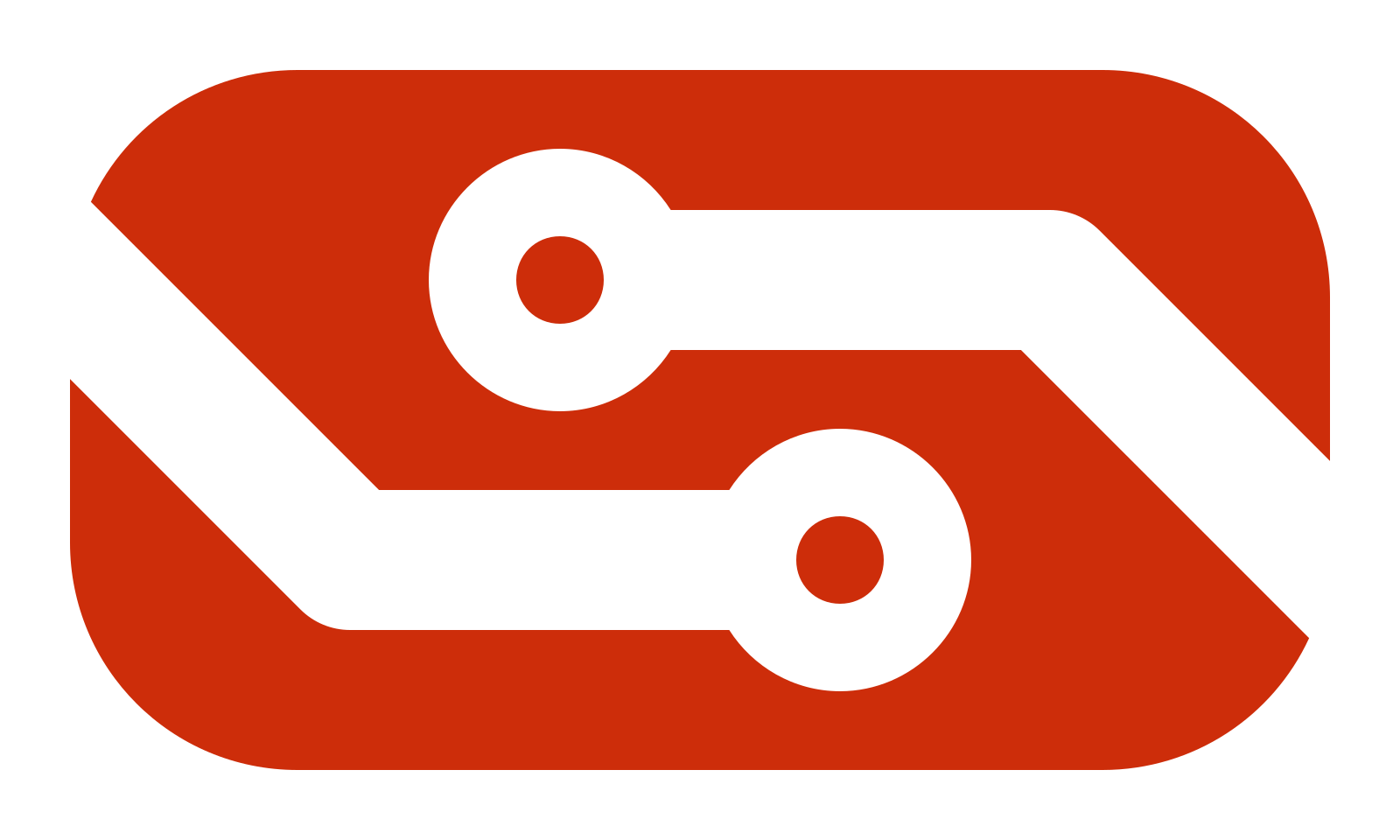# Competition Theme Complexity

Read carefully before voting, there are THREE separate polls.
These polls are to gage what complexity the average competitor is ok with so we don’t have something too easy or too hard.

In the poll BELOW pick the builds that are TOO EASY in your opinion

• CCA ALU
• Fibonacci Circuit
• Barrel Shifter
• Multiplier
• Collatz Circuit (A circuit that takes an input and performs the steps of the Collatz Conjecture until the value reaches 1)
• Sequential Squares Circuit (A circuit that outputs 1, 4, 9, 16, 25…)
• Sorter Circuit
• Line Drawer
• Floating Point Arithmetic Circuit (Addition, Subtraction, etc with Floating Point inputs and outputs)

0 voters

In the poll BELOW pick the builds that are TOO HARD in your opinion

• CCA ALU
• Fibonacci Circuit
• Barrel Shifter
• Multiplier
• Collatz Circuit (A circuit that takes an input and performs the steps of the Collatz Conjecture until the value reaches 1)
• Sequential Squares Circuit (A circuit that outputs 1, 4, 9, 16, 25…)
• Sorter Circuit
• Line Drawer
• Floating Point Arithmetic Circuit (Addition, Subtraction, etc with Floating Point inputs and outputs)

0 voters

In the poll BELOW pick the builds that are REASONABLE COMPLEXITY in your opinion

• CCA ALU
• Fibonacci Circuit
• Barrel Shifter
• Multiplier
• Collatz Circuit (A circuit that takes an input and performs the steps of the Collatz Conjecture until the value reaches 1)
• Sequential Squares Circuit (A circuit that outputs 1, 4, 9, 16, 25…)
• Sorter Circuit
• Line Drawer
• Floating Point Arithmetic Circuit (Addition, Subtraction, etc with Floating Point inputs and outputs)

0 voters

2 Likes

To be clear, these aren’t actual themes you’re picking, just examples to gage complexity

2 Likes

FPU Competition wen

2 Likes

Ah yes I know a theme where no one gets the money

Build a piston door

2 Likes

I’d like to note that the perceived complexity of sequential squares may not be very accurate - it would be reasonable to assume at first glance that one would need a multiplier, but infact it can be done with only a couple of adders, thus people may perceive it as way harder than it really is. For example looking at the results its clear that people perceive sequential squares as being harder than a multiplier but this is not the case (as long as you know how).

Just gotta add consecutive odd numbers